File Exchange Try Origin for Free
The Origin Forum
Home | Profile | Register | Active Topics | Members | Search | FAQ | Send File to Tech support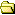All Forums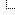Origin Forum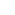Origin Forum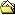Integrating log-log data ?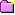New Topic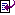Reply to Topic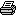Printer Friendly
Author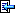Topic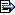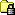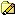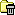USA
0 Posts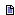Posted - 02/03/2000 :  12:51:00 PM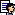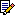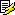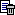I have an x-y dataset (x=frequency, y=power spectral density). The data is log-log, i.e.,when plotted on log-log paper, the data points are connected by straight lines. I need to calculate the area under the curve. How does one do this.Using the Origin Integrate function does not work. This calculates the area for data as if the data were linear, even when I use it on a log-log plot.Is there a simple way to integrate log-log data ?Thanks,Andreas

USA
150 PostsPosted - 02/04/2000 :  8:02:00 PMHi Andreas,The area returned by the Origin Integrate function is correct. You have changed the scale on which the data is displayed but you have not changed the data. The integration of the curve returns the actual area under the curve independent of how the data is displayed. The integration of the function f(x)=10 from 1 to 100 is 990 whether the plot is displayed on a linear-linear or a log-log scale. Integration does not return the area of the "paper" under the displayed curve it returns the area under the function.One way to calculate the area of the paper under the displayed curve on a log10-log10 scale would be to log the data before plotting, plot the logged data (in linear space), and then integrate. To log the data click the new column button twice to add two new columns, set the column designation of the first new column to X (right click on the column name and select Set as X), right click on each new column and select Set Column Values, and then enter a formula similar too Col(C)=log(Col(A)) (depending on your column names), and then click OK. Plot the logged data in the new columns and then integrate as normal...the value returned will then be akin to the area of the paper under the original data when plotted on a log10-log10 scale.I hope this is helpful.-Gary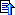USA
0 PostsPosted - 02/04/2000 :  8:55:00 PMHi Gary,I could not get your suggestion to work correctly. Let me explain the problem in a bit more detail.Lets take the following data set:Freq. (xi) PSD (yi)20 0.01270 0.115300 0.1152000 0.006When plotted on log-log paper, intermediate points would be on a straight line connecting the given points. (The given numbers are not logged before plotting).Using the integrate function for the original data gives an area of 132.475."Logging" the data givesC(x) C(y)1.30103 -1.920821.8451 -0.93932.47712 -0.93933.30103 -2.22185and an integral of -2.67396. That is notcorrect since the area on the paper under alog-log plot can not be negative (y-values are always > 0).For the exact solution one can calculate the area of the individual segments using the following equations:slope = (log(y2))-log(y1))/(log(x2)-log(x1))if slope = -1area = x1 * y1 * LN[x2 / x1]elsearea = y1/(slope+1)*((x2/x1)^slope * x2 - x1)If you do this for the three segments of theabove area you get approx 69.7.Do you have any idea how to get this with Origin ?-------Just as a side note:The data I have is from a random vibration test. The x-values are the frequencies. The y-values are the power spectral density given in g^2/Hz (g = gravitational acceleration). What I need to calculate is the root mean square acceleration for the given data.USA
150 PostsPosted - 02/07/2000 :  3:19:00 PMHi Andreas,Thanks for the second post. I verified everything that you reported although I am sure that there is no bug involved...Origin is mathematically correct in everything that was done.So, I copy/pasted your raw (un-logged data) into a worksheet named Data1 containing columns A(X) and B(Y) and ran the following script:`get Data1_A -e npts;area=0;for(ii=2;ii<=npts;ii++) { yy1=Data1_B[ii-1]; xx1=Data1_A[ii-1]; yy2=Data1_B[ii]; xx2=Data1_A[ii]; slope=(log(yy2)-log(yy1))/(log(xx2)-log(xx1)); if(slope==-1) {temp=xx1*yy1*ln(xx2/xx1);} else {temp=yy1/(slope+1)*((xx2/xx1)^slope*xx2-xx1)}; area=area+temp;};area=;`The script is not pretty but it works. It essentially implements your formula. We generally don't provide complete scripts but this was easy enough to do given your formula. All I did was add the for loop and change your variable names. X1, X2, Y1, and Y2 are reserved system variables in Origin.The script typed out the correct result in the script window:AREA=69.65484855105967I hope this is helpful.-Gary [This message has been edited by Gary Lane (edited 02-07-2000).]USA
0 PostsPosted - 02/08/2000 :  8:23:00 PMHi Gary,thanks for the reply. I'll try the script as soon as I find a spare minute.You wrote:"I verified everything that you reported although I am sure that there is no bug involved...Origin is mathematically correct in everything that was done."I did not intend to raise any doubts about the origin integration function. The integration function does exactly what it is supposed to do. Only for my problem the trapezoidal rule of integration was not the appropriate rule to use. If one generated an increasing number of intermediate points (between the points I had given in my previous mail), then the integration using the trapezoidal rule would converge to the result the script gives.So,again, I did not intent to raise any doubts about the origin integration function in any way.Cheers,AndreasUSA
1 PostsPosted - 03/14/2014 :  12:13:19 PMAndreas, Gary, or anyone,I am facing a similar problem that is described here in 2000. Would you please explain to me how you come up with these equations:For the exact solution one can calculate the area of the individual segments using the following equations:slope = (log(y2))-log(y1))/(log(x2)-log(x1))if slope = -1area = x1 * y1 * LN[x2 / x1]elsearea = y1/(slope+1)*((x2/x1)^slope * x2 - x1)What is the units for area?If my y axis values are already expressed is log units (dBW), is it correct to use the following equations:slope = (y2-y1)/(log(x2)-log(x1))if slope = -1area = x1 * 10^(y1/10) * LN[x2 / x1]elsearea = 10^(y1/10)/(slope+1)*((x2/x1)^slope * x2 - x1)Sincerely appreciate any help you can provide.KhanhUSA
1 PostsPosted - 12/18/2014 :  2:19:58 PMThe units for log-log plots are units of x * units of y (as is with all areas under curves). Log-log plotting doesn't change that. dB transformations will, so be careful how you proceed with your dB converted data.To me, it looks like your conversion is correct, but you can judge that from the derivation below.The derivation of the formula is to determine the original function of the curve, which is y=cx^m for log-log straight lines between two points (see http://en.wikipedia.org/wiki/Log-log_plot).Integrating y, you have c/(m+1)*x^(m+1)+constant (not needed since we're talking definite integrals in the long run)To determine c, you solve the original equation and get c=y1/(x1)^m.Substitute in c and you have integral=y1/(m+1)*(x1^-m)*x^(m+1)+const.Calculating the definite integral over the two endpoints, you haveA=y1/(m+1)*(x1^-m)*(x2^[m+1]-x1^[m+1]). Using some log rules,logA=log{y1/[m+1]*[x2^(m+1)/x1^m-x1^(m+1)/x1^m]}logA=log[y1/(m+1)]+log[(x2/x1)^m*x2-x1]Then reversing the log functionA=y1/(m+1)*[x2*(x2/x1)^m-x1]To get anything useful (I'm assuming you want W*something units, I would suggest converting back before you start and use the original formula so that your units are correct) Edited by - postguard64 on 12/18/2014 2:25:16 PMTopicNew TopicReply to TopicPrinter Friendly Jump To: Select Forum Origin Forum       Origin Viewer Forum       Origin Forum Origin Forum for Programming       Forum for Python       LabTalk Forum       Forum for Origin C       Forum for Automation Server/COM and LabVIEW Origin中文论坛       Origin 中文论坛 (Chinese Origin Forum) Japanese Origin Forum       Origin日本語フォーラム (Japanese Origin Forum) Origin on Linux       The Origin on Linux Forum Private Forums       Distributor Forum  -------------------- Home Active Topics Frequently Asked Questions Member Information Search Page
 The Origin Forum © 2020 Originlab Corporation